×

Search anything:

# NullPointerException in Java

#### Java Software EngineeringGet this book -> Problems on Array: For Interviews and Competitive Programming

## Introduction

Any developer beginning their Java programming journey will inevitably come across a certain error: the NullPointerException. The NullPointerException is one of the most common errors encountered by beginner programmers when dealing with objects in Java.

So what exactly is a NullPointerException? How exactly does this error happen? What are some ways to avoid these errors?

To answer these questions, we will discuss the following concepts:

• Primitive vs. reference types
• The null value
• The NullPointerException error
• Strategies in preventing NullPointerExceptions

## Primitive vs. Reference Types

In order to properly discuss NullPointerExceptions, we must first review the difference between primitive and reference types.

Primitive data types are data types that have built-in values determined by the Java programming language. When assigned a primitive-type value, a variable stores the actual value itself. For instance, the statement:

``````int num = 0;
``````

stores the integer value "0" into the primitive type variable "num".

Primitive data types are usually denoted in lowercase letters, such as:

• int
• double
• boolean
• float
• byte
• char

Reference types, by contrast, store the addresses of objects, not actual values. When invoked in Java, these variables reference the memory address for an object. One example is the statement:

``````String s = "Hello, World!";
``````

In this case, the variable s is a reference type, pointing to the memory address of the string literal "Hello, World!".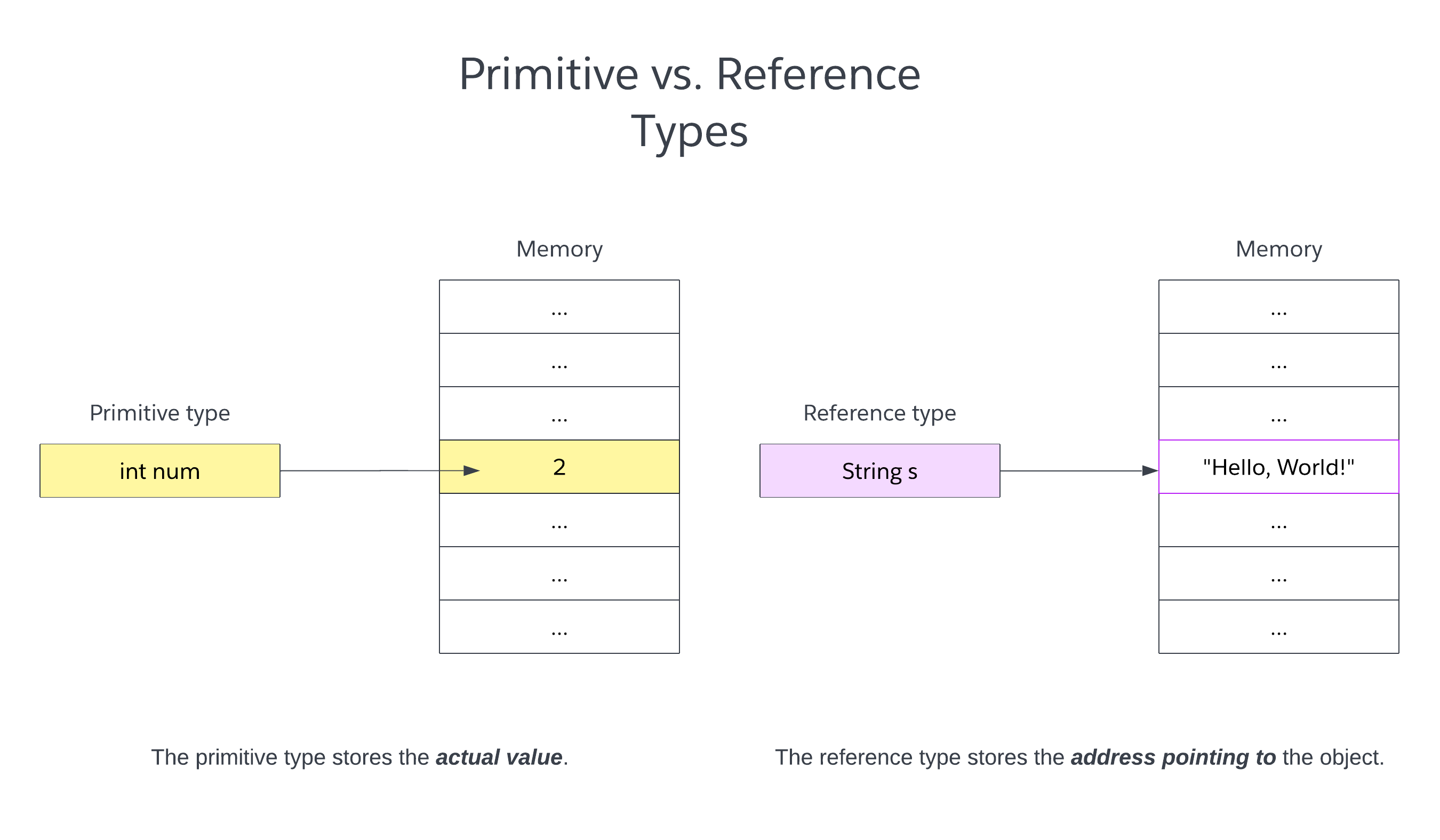Reference data types are usually classes, either user-defined or built into the Java programming language. Examples include:

• String
• ArrayList
• BigInteger
• Arrays (of primitive or reference type)

So what does this have to do with the NullPointerException?

Unlike primitive types, reference types can be declared null.

## The null value

The null value in Java represents an absence of a value.

Null values can be assigned to reference types in assignment statements:

``````String s = null;
``````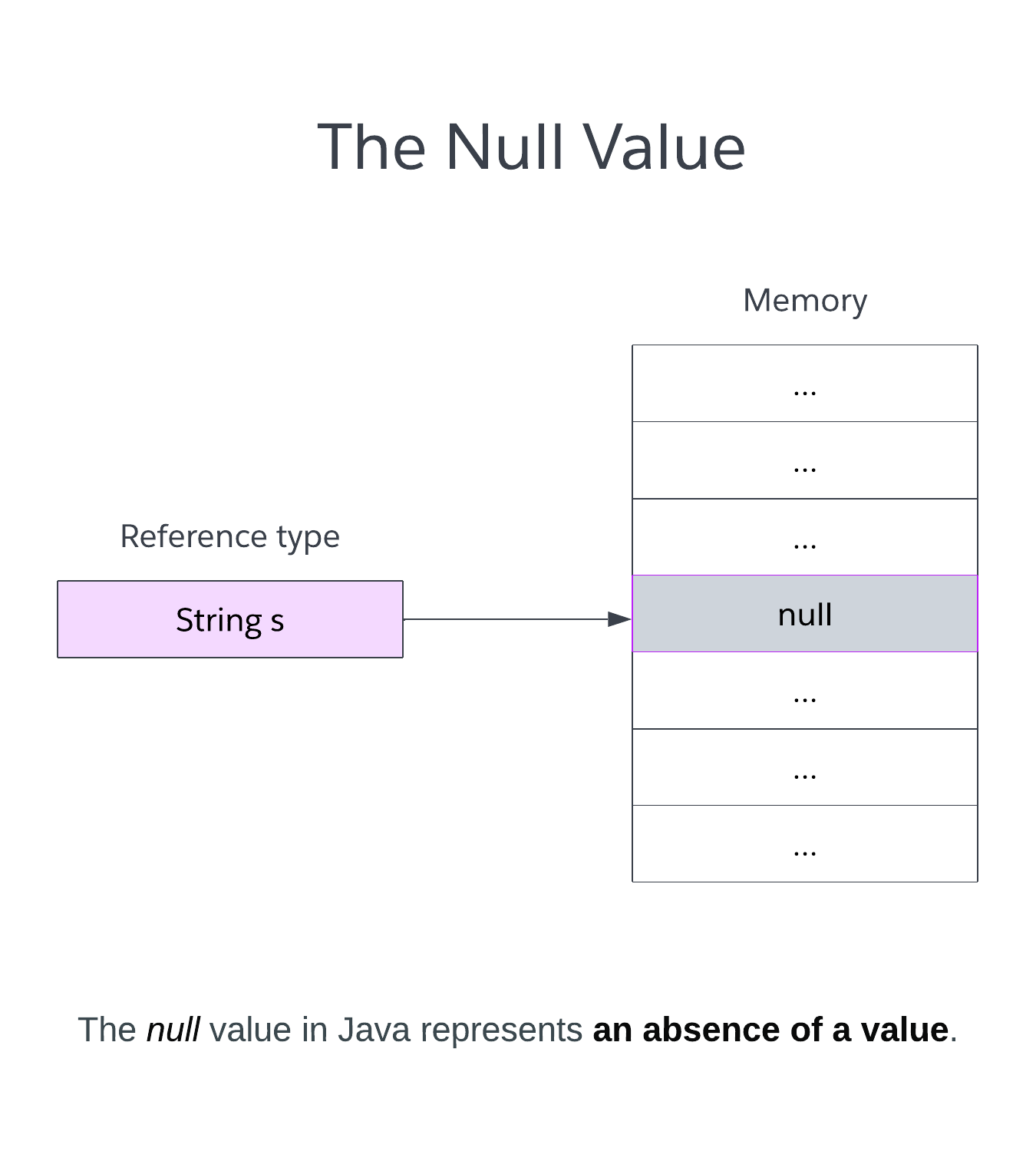Assigning a value null to a variable is essentially saying, "I've made a variable that points to nothing."

As mentioned before, arrays are reference types. Therefore, variables pointing to arrays can also be designated null:

``````int[] nums = null;
``````

## NullPointerException

With the concepts of primitive/reference types and the null value discussed, we can finally now talk about NullPointerExceptions.

A NullPointerException happens when a reference variable is used or accessed that is actually null.

This can happen in several ways, including:

• Calling a method associated with the null object
• Accessing or modifying properties of the null object

One of the simplest ways a NullPointerException can happen is the following scenario:

### Example 1:

``````public class Main {
public static void main(String[] args) {
// object initialized to "null"
String s = null;

// the line attempts to get the
// first character of the string,
// resulting in a NullPointerException
System.out.println(s.charAt(0));
}
}
``````

If you were to compile and run the above code, the terminal output will look something like this:

``````Exception in thread "main" java.lang.NullPointerException: Cannot invoke "String.charAt(int)" because "<local1>" is null
at Main.main(Main.java:9)
``````

The error tells us Main.java:9 (line 9 in the code) tries to invoke the charAt String method on the null object s. Because the string object was initialized with a value of null, the line will cause a NullPointerException.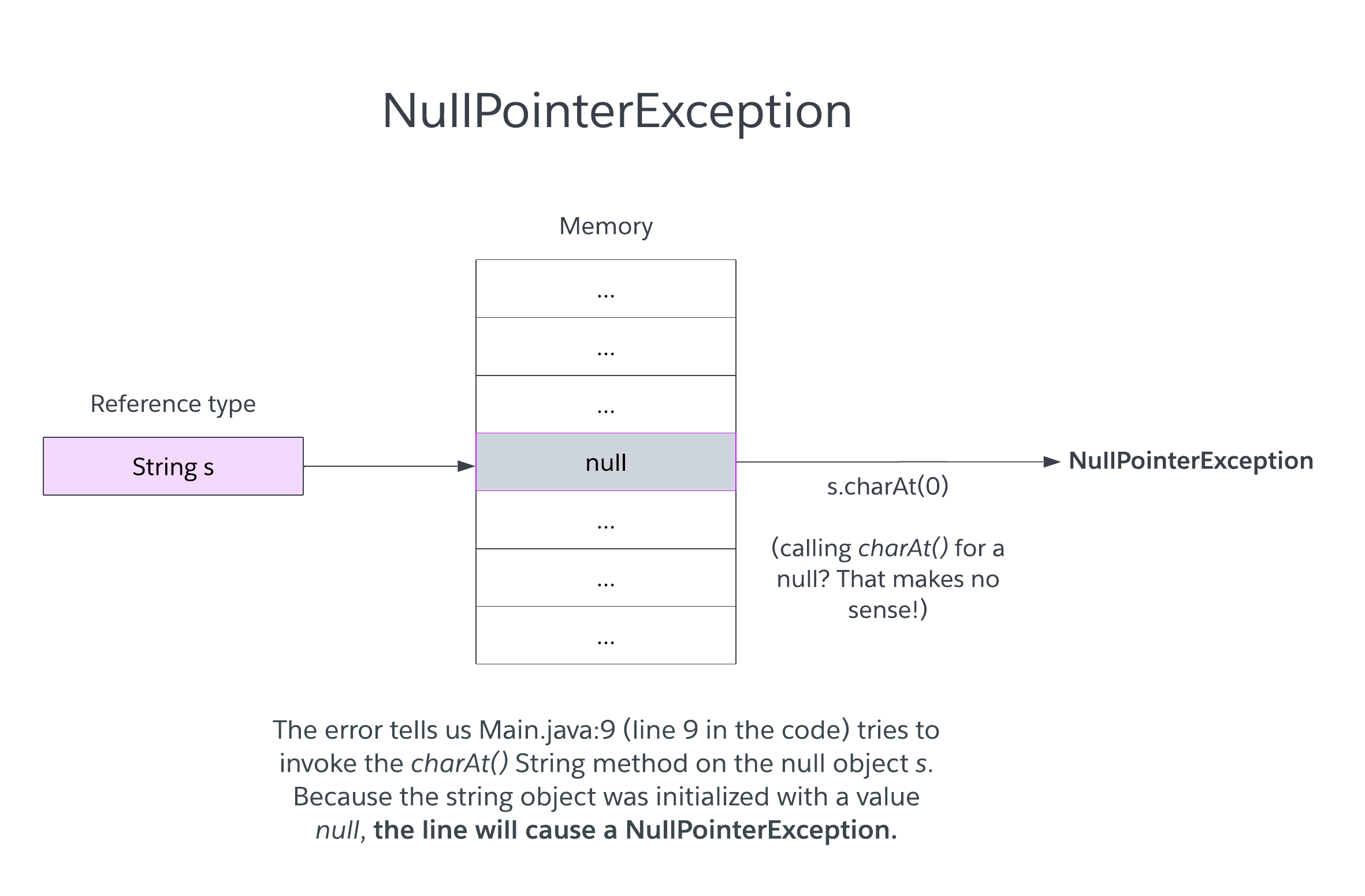Similarly, if a null value is passed into a function that assumes it's an object, that can also produce a NullPointerException.

### Example 2:

``````import java.util.ArrayList;

public class Main {
/**
* Puts a number into the list if it
* @param nums - an ArrayList of numbers
* @param number - some intger to put into the list
*/
private static void putNumber(ArrayList<Integer> nums, int number) {
// checks if number exists in list,
// then puts the number into the
// list
if (!nums.contains(number)) {
}
}

public static void main(String[] args) {
// the ArrayList is actually null
ArrayList<Integer> nums = null;

putNumber(nums, 10);
}
}
``````

Compiling and running the code will result in the following error:

``````Exception in thread "main" java.lang.NullPointerException: Cannot invoke "java.util.ArrayList.contains(Object)" because "<parameter1>" is null
at Main.putNumber(Main.java:14)
at Main.main(Main.java:23)
``````

The error tells us that the error originated at line 14 of the code (which belongs to the function putNumber() we defined). Because we passed a null object as a parameter, the function throws an error when it tries to invoke add() on nums.

However, things are not always so simple, such as this code:

### Example 3:

``````import java.util.Random;

public class Main {
/**
* Checks if the numbers are all odd.
* @param nums - the array holding the numbers.
* @return true if all numbers are odd, false otherwise.
*/
private static boolean isAllOdd(int[] nums) {
// if any of the numbers has a remainder
// of 0 when dividing by 2 (aka it's even),
// then return false (they're not all odd)
for (int i = 0; i < nums.length; i++) {
if (nums[i] % 2 == 0) {
return false;
}
}

// return true (they're all odd numbers)
return true;
}

/**
* Function intended to initialize an array
* of size "size" with random numbers.
* @param nums
* @param size
*/
private static void initializeNums(int[] nums, int size) {
// initialize the nums array, setting it from
// null to an actual object
nums = new int[size];

// initialize random object to generate random
// integers
Random random = new Random();

// generate numbers until the nums array
// is filled with integers
for (int i = 0; i < nums.length; i++) {
nums[i] = random.nextInt();
}
}

public static void main(String[] args) {
// initialized to null
int[] numbers = null;

// initialized to some array of integers
initializeNums(numbers, 10);

// it should print either "true"
// or "false", right???
// right????????
System.out.println(isAllOdd(numbers));
}
}
``````

At first glance, the code seems completely fine. We seem to have taken care of the null issue by initializing the numbers array to an actual array of 10 numbers. Thus, after compiling and running the code, the terminal should be able to print either "true" or "false".

Except it doesn't:

``````Exception in thread "main" java.lang.NullPointerException: Cannot read the array length because "<parameter1>" is null
at Main.isAllOdd(Main.java:13)
at Main.main(Main.java:55)
``````

It's not immediately clear why this example outputs a NullPointerException. After all, the code clearly passes the reference numbers to the initializeNums() method.

That is because Java passes by value, not by reference.

What does this mean?

It means that, for a given function (take, for instance, our initializeNums() function), Java actually allocates new memory for the function parameters (it creates space for nums and size).

That's fine for the size parameter because we only really care about the actual value anyway. However, the nums parameter is the one being initialized, NOT the the original numbers array. Therefore, since numbers is still null, calling isAllOdd() on numbers will cause a NullPointerException.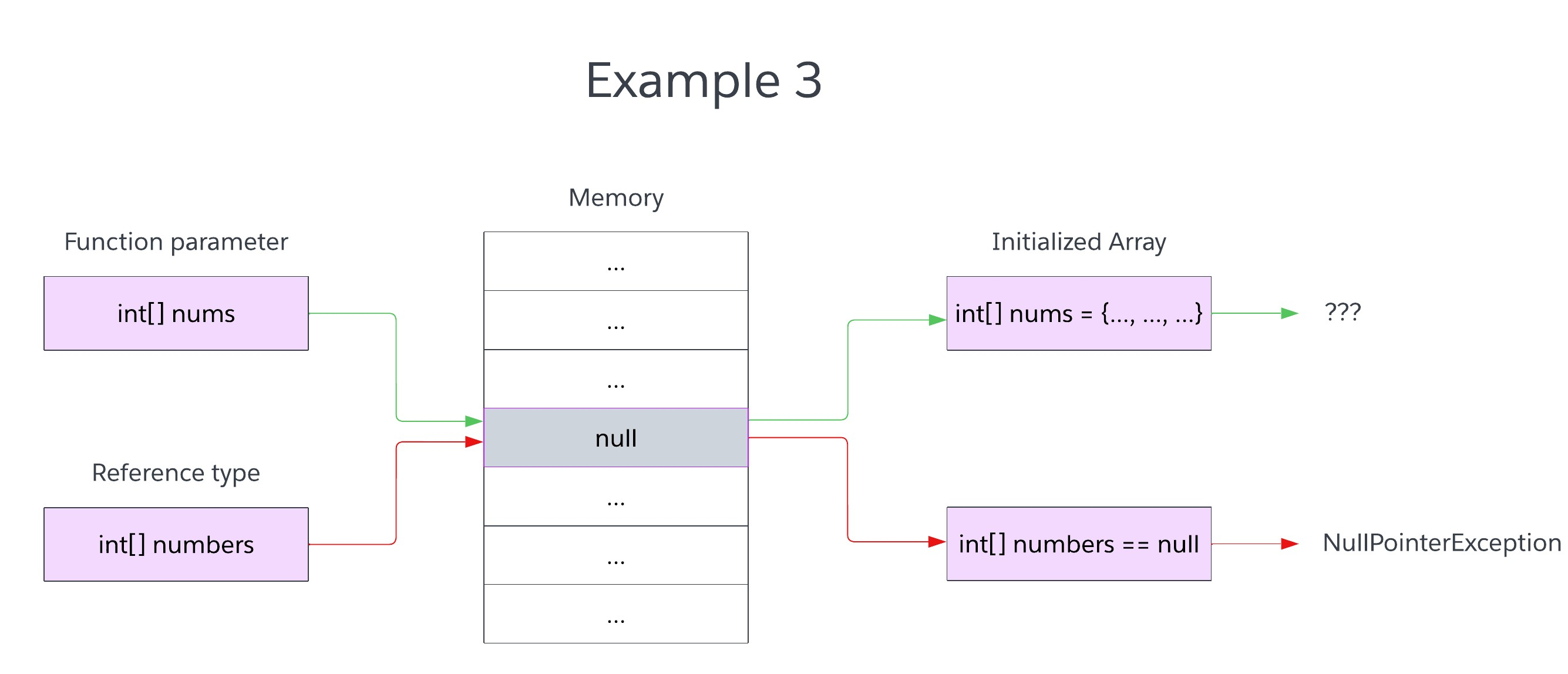### Example 4

``````public class Main {
public static void main(String[] args) {
// initializes an array of empty strings, right?
String[] array = new String;

// an entry in the array should be an empty string,
// right?
System.out.println(array.equals(""));
}
}
``````

At first glance, the code seems to look fine. Since array was initialized as a String array of size 10, each entry should have an empty string. Therefore, comparing the first entry in the array with an empty string should print "true", right?

No.

``````Exception in thread "main" java.lang.NullPointerException: Cannot invoke "String.equals(Object)" because "<local1>" is null
at Main.main(Main.java:8)
``````

Each entry in the array is a reference type. Entries in primitive type arrays are automatically initialized to some value (by virtue of primitive data types being pre-defined); however, entries in reference type arrays are simply given null values. As a result, an initialized array of reference types will actually contain entries with all null values.

## Strategies in Dealing With NullPointerExceptions

With that said, what are some possible ways a programmer can catch null values where they shouldn't be?

First, we can implement checks for any functions that make use of reference types.

Take, for instance, Example 2. We can add if statements before the putNumber() function that check whether or not any reference type parameters are null:

### Make use of null checks

``````import java.util.ArrayList;

public class Main {
/**
* Puts a number into the list if it
* @param nums - an ArrayList of numbers
* @param number - some intger to put into the list
*/
private static void putNumber(ArrayList<Integer> nums, int number) {
// checks if the list is null. If yes,
// then return without doing anything
// (or handle it some other way)
if (nums == null) {
return;
}

// checks if number exists in list,
// then puts the number into the
// list
if (!nums.contains(number)) {
}
}

public static void main(String[] args) {
// the ArrayList is actually null
ArrayList<Integer> nums = null;

putNumber(nums, 10);
}
}
``````

If we run the code, the NullPointerException would be avoided, or a programmer may choose to do something else when a null object is passed (i.e. they may throw some kind of exception).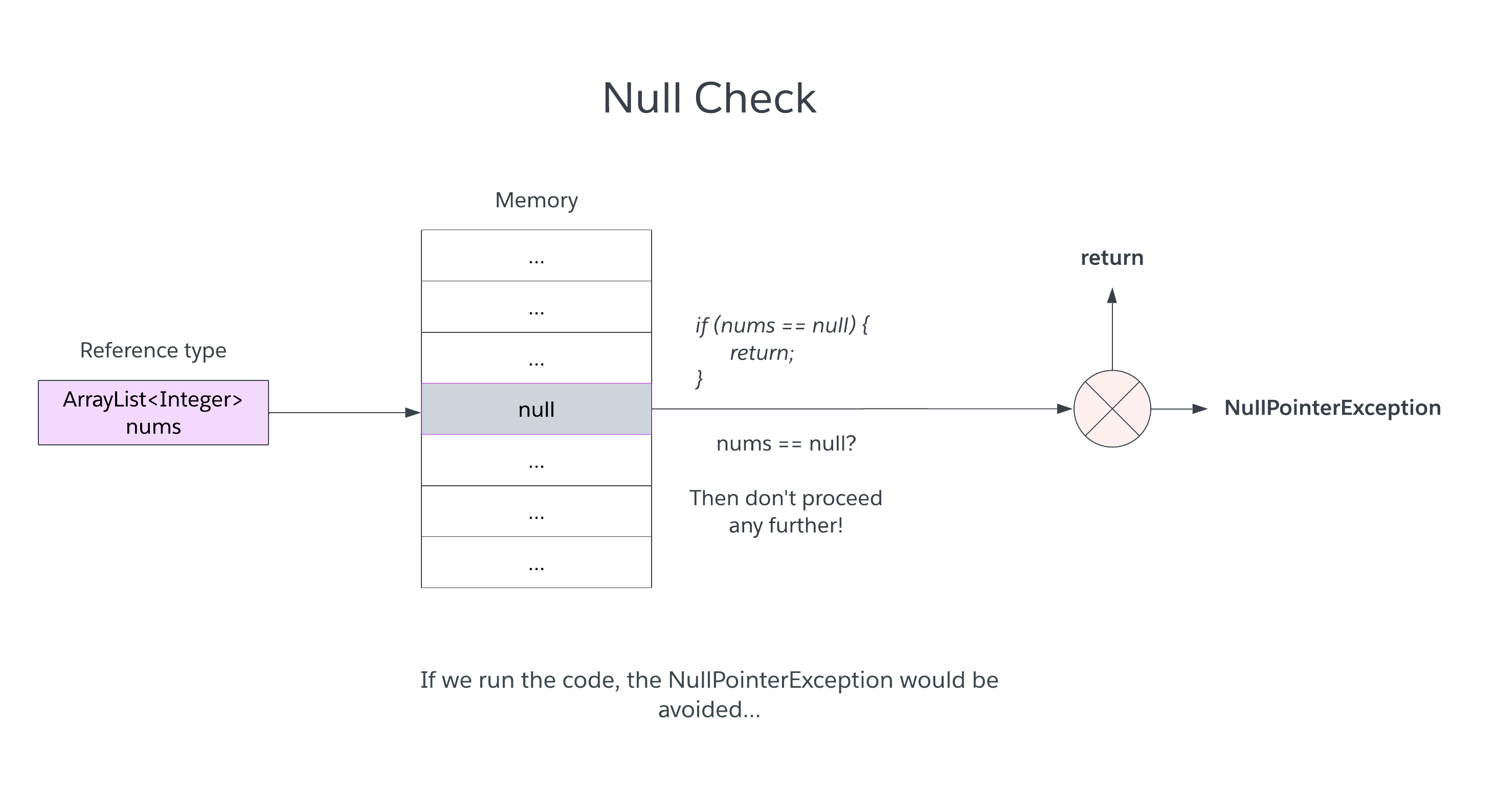Another way to avoid NullPointerExceptions is to make use of the function Object.requireNonNull(). This requires any object passed into the method to be not null; otherwise, the method will throw a NullPointerException.

### Objects.requireNonNull()

``````import java.util.ArrayList;
import java.util.Objects;

public class Main {
/**
* Puts a number into the list if it
* @param nums - an ArrayList of numbers
* @param number - some intger to put into the list
*/
private static void putNumber(ArrayList<Integer> nums, int number) {
// checks if the list is null. If yes,
// then the method will throw a NullPointerException.
Objects.requireNonNull(nums);

// checks if number exists in list,
// then puts the number into the
// list
if (!nums.contains(number)) {
}
}

public static void main(String[] args) {
// the ArrayList is actually null
ArrayList<Integer> nums = null;

putNumber(nums, 10);
}
}
``````

When the null nums list is passed into Objects.requireNonNull() (and an exception is thrown), the programmer can pinpoint where the exception took place and find out where the null value originated. Null checks such as these are especially invaluable for Java newcomers who have yet to encounter a subtle bug like the one in Example 3.

Programmers can also take preventative measures while coding to ensure that a reference variable is initialized correctly. Take, for instance, Example 3. Since we now know that the code won't work, what can we do?

One solution is this: instead of initializing the array inside the function, we can return the initialized array and set the original array to said returned value, like so:

``````import java.util.Random;

public class Main {
/**
* Checks if the numbers are all odd.
* @param nums - the array holding the numbers.
* @return true if all numbers are odd, false otherwise.
*/
private static boolean isAllOdd(int[] nums) {
// if any of the numbers has a remainder
// of 0 when dividing by 2 (aka it's even),
// then return false (they're not all odd)
for (int i = 0; i < nums.length; i++) {
if (nums[i] % 2 == 0) {
return false;
}
}

// return true (they're all odd numbers)
return true;
}

/**
* Function intended to initialize an array
* of size "size" with random numbers.
* @param nums
* @param size
* @return int[] of initialized numbers.
*/
private static int[] initializeNums(int size) {
// initialize the nums array, setting it from
// null to an actual object
int[] nums = new int[size];

// initialize random object to generate random
// integers
Random random = new Random();

// generate numbers until the nums array
// is filled with integers
for (int i = 0; i < nums.length; i++) {
nums[i] = random.nextInt();
}

return nums;
}

public static void main(String[] args) {
// initialized to null
int[] numbers = null;

// the original array is set equal to
// the initialized array
numbers = initializeNums(10);

// now it should print "true" or
// "false"
System.out.println(isAllOdd(numbers));
}
}
``````

This in turn generates either "true" or "false", depending on what numbers your program chose randomly. Regardless, the important part is that the NullPointerException was avoided successfully.

In addition, every entry in a reference-type array should be initialized, in addition to the array itself. Take, for instance, a reworked Example 4:

``````public class Main {
public static void main(String[] args) {
// initializes a String array of size 10
String[] array = new String;

// initializes EACH entry of the String array
for (int i = 0; i < array.length; i++) {
array[i] = "";
}

// now, the console should print "true"
System.out.println(array.equals(""));
}
}
``````

Initializing every entry in the String array ensures that the entries are not null. In turn, the NullPointerException is avoided.

NOTE: Nowadays, development environments, such as Visual Studio Code and IntelliJ, have features that can detect possible NullPointerExceptions. Often, a certain variable or line of code will be highlighted with a warning saying something along the lines of: "this may cause a NullPointerException."

## Conclusion

So what have we learned today?

• Primitive types store actual values, whereas reference types store addresses to objects.
• Reference types include items such as built-in classes (String, ArrayList, etc.), uesr-defined classes, and arrays of values, primitive or reference.
• A null value is an absence of value assigned to a reference variable.
• Primitive types cannot have value null; by contrast, reference types can have the null value.
• A NullPointerException takes place when a reference variable is used or accessed that has actually been assigned null.
• Programmers can use a variety of strategies to deal with NullPointerExceptions, such as null checks, Object.requireNonNull(), and other conscientious programming practices.

## Question 1

#### Which of the following is NOT an example of a reference type?

String
double
Primitive type array
ArrayList
The *double* type in Java is a primitive type. Strings, Arrays, and ArrayLists are reference types.

## Question 2

#### True or False? Primitive types can have value null

True
False
Only reference types can be initialized to null.

With this article at OpenGenus, you must have the complete idea of NullPointerException in Java.

#### Yejoong (Paul) Kim

Yejoong (Paul) Kim is a Computer Science student at Princeton University and is a Software Developer, Intern at OpenGenus.